# Analyze T-Coil Circuit

This example shows how to use the Linear Circuit Wizard block to analyze a T-coil impedance matching circuit and generate S-parameter data from the result.

The T-coil circuit is used in many applications to impedance match a capacitive load, such as an electrostatic discharge (ESD) protection device, over as broad a frequency band as possible , . You need to include the effects of a T-coil circuit in the model of any broadband or microwave channel that uses it. Depending on the application, you may insert the transfer function of the circuit into a model of the channel or insert the S-parameters of the circuit into the RF analysis of the channel.

This example analyzes the T-coil circuit shown in this schematic diagram. Capacitor Ce is the capacitor to be impedance matched . The schematic includes the equivalent circuits for two S-parameter ports. For more about S-parameter ports, see the S-Parameters section of the example.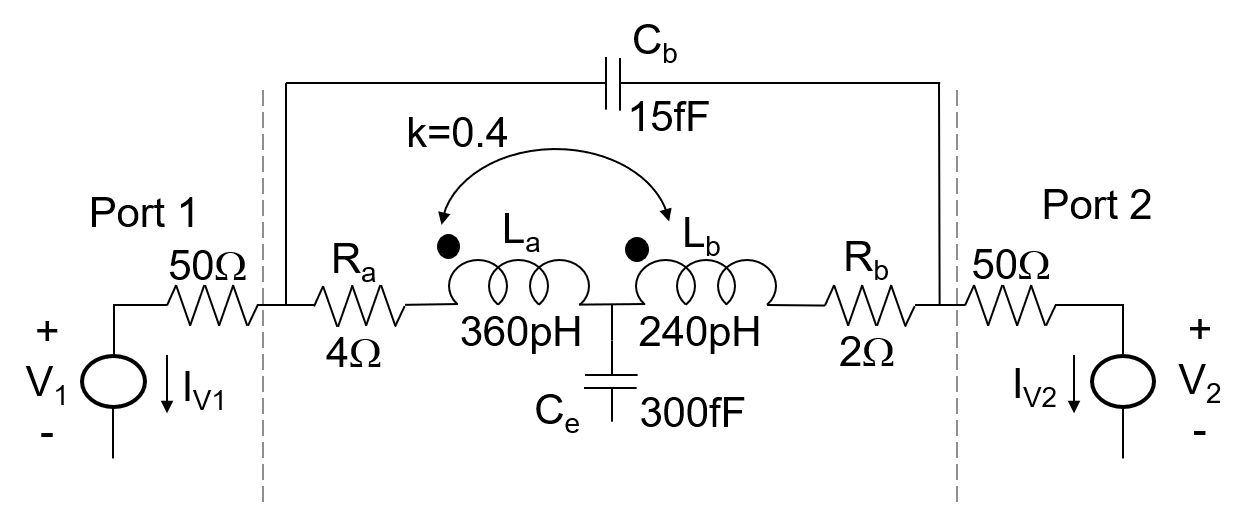The file TCoil.sp contains the SPICE netlist for the T-coil circuit.

* SPICE netlist for T-coil example * The circuit itself Ra 2 3 4 La 3 4 360e-12 Cb 2 6 15e-15 Kab La Lb 0.4 Ce 4 0 300e-15 Lb 4 5 240e-12 Rb 5 6 2 * Create an S-parameter port by driving with a voltage source * and load resistor, and then measure the voltage and current * at the circuit end of the load resistor. * * Port 1 definition V1 1 0 R1 1 2 50 .print V(2 0) I(V1) * Port 2 definition V2 7 0 R2 6 7 50 .print V(6 0) I(V2) .end

Open the TCoil model. The model contains the Linear Circuit Wizard that you can use to create Linear Circuit blocks for the T-coil circuit and a baseline circuit without impedance matching. The model also contains a test setup used to compare the pulse response of the T-coil circuit to the baseline circuit. The time scope from the DSP System Toolbox library is used rather than the scope from the Simulink library because its rendering of the waveforms is smoother in this application.

open_system('TCoil.slx');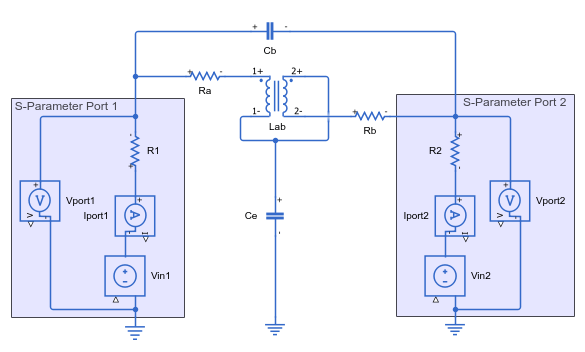Select the Linear Circuit Wizard block. In the block mask or the Property Inspector, set the Circuit design name to 'T-Coil' and the Block name to 'with T-coil'.

Parse the SPICE netlist for the T-coil circuit by clicking the Parse netlist file and redefine ports button. The Port Definition and Device Noise Generators tabs become visible in the block parameter dialog box. If desired, review the port settings and device noise settings.

Display the frequency response of the T-coil circuit by clicking the Plot transfer functions button.Build a Linear Circuit block for the T-coil circuit by clicking the Build/modify block button. Connect the block into the lower channel in the model. The active input port is V1 and the active output port is Vprint3.This example compares the T-coil circuit against a baseline circuit without impedance matching. The baseline circuit is a shunt capacitor connected to two S-parameter ports. The file NoTCoil.sp contains the SPICE netlist for this circuit.

* SPICE netlist for T-coil example * The load parasitic capacitance without matching circuit Ce 4 0 300e-15

* Create an S-parameter port by driving with a voltage source * and load resistor, and then measure the voltage and current * at the circuit end of the load resistor. * * Port 1 definition V1 1 0 R1 1 4 50 .print V(4 0) I(V1) * Port 2 definition V2 7 0 R2 4 7 50 .print V(4 0) I(V2) .end

Return to the Linear Circuit Wizard block. In the block mask or the Property Inspector, set the Circuit design name to 'Shunt C', the Block name to 'no T-coil', and the Netlist file name to 'NoTCoil.sp'. Parse the netlist for the shunt capacitor circuit by clicking the Parse netlist and redefine ports button.

Display the frequency response of the shunt capacitor circuit by clicking the Plot transfer functions button. The response of the shunt capacitor falls off much faster at high frequencies than the response of the T-coil circuit.Build a Linear Circuit block for the shunt capacitor by clicking the Build/modify block button. Connect this block into the upper channel in the model. The active input port is V1 and the active output port is Vprint3.Run the simulation. In this scope display, the yellow trace is the pulse response of the shunt capacitor without impedance matching and the blue trace is the pulse response of the T-coil circuit.

Although the T-coil circuit introduces a little delay, the resulting pulse response is more desirable for data transmission, with a flat top and more compact rising and falling edges.### S-Parameters

The Linear Circuit Wizard defines ports as being either input or output and as being either voltage or current. In contrast, S-parameters are expressed in terms on incoming waves and outgoing waves at each port, based on an assumed transmission line impedance at each port. You therefore need some specific assumptions and some conversion to obtain S-parameters for a linear circuit.

The example makes these assumptions:

1. Each port is connected to a source/load impedance of Z0 (typically 50 Ω).

2. Each port is driven by an independent voltage source driving a resistor whose value is equal to the source/load impedance. This voltage source is an input voltage port.

3. The voltage at each port is available as an output. This is a voltage output port.

4. The current flowing through each source/load resistor is available as an output. This is a current output port. In the context of the SPICE netlist, this current is measured by the independent voltage source that defines the input voltage port.

Given these assumptions, you can transform the port node voltages and currents into incoming and outgoing waves to produce S-parameters. Given incoming wave voltage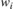and outgoing wave voltage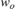at a port with characteristic impedance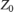, and remembering that the port current is defined as an output current of the circuit, the port voltage and port current are: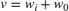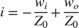Solving these equations yields the resulting waves: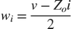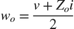The S parameter matrix is then a matrix of outgoing wave amplitudes divided by incoming wave amplitudes.

The netlist TCoil.sp satisfies this set of assumptions for S-parameter Port 1 through the following group of statements:

* Port 1 definition V1 1 0 R1 1 2 50 .print V(2 0) I(V1)

V1 defines the voltage input port and R1 is the source/load resistor. The .print statement defines a voltage output port from node 2 to the return node and a current output port whose output is the current flowing through the voltage source V1.

A similar set of statements defines S-parameter Port 2.

You can calculate the S-parameter data by first defining a desired frequency scale and then using the generateSParameterData helper function. Parse the netlist of interest and then click the Export poles and zeros button. Obtain the S-parameter data from DC to 100 GHz in 1 GHz steps.

freq = (0:100)*1e9; [data,errmsg] = generateSParameterData('TCoil/Linear Circuit Wizard',freq);

The resulting data structure is directly compatible with the S-parameters object and the rfwrite function in the RF Toolbox™.### References

1. Razavi, Behzad. "The Bridged T-Coil [A Circuit for All Seasons]." IEEE Solid-State Circuits Magazine 7, no. 4 (Fall 2015): 9–13. https://doi.org/10.1109/MSSC.2015.2474258.

2. Ross, Bob. "T-Coil Topics." DesignCon IBIS Summit, Santa Clara, Ca. February 3, 2011. https://ibis.org/summits/feb11/ross.pdf

3. Kossel, Marcel, Christian Menolfi, Jonas Weiss, Peter Buchmann, George von Bueren, Lucio Rodoni, Thomas Morf, Thomas Toifl, and Martin Schmatz. "A T-Coil-Enhanced 8.5Gb/s High-Swing Source-Series-Terminated Transmitter in 65nm Bulk CMOS." In 2008 IEEE International Solid-State Circuits Conference - Digest of Technical Papers, 110–599, 2008. https://doi.org/10.1109/ISSCC.2008.4523081.